{{announcement.body}}
{{announcement.title}}

# Object Detection Tutorial in TensorFlow: Real-Time Object Detection

DZone 's Guide to

# Object Detection Tutorial in TensorFlow: Real-Time Object Detection

### In this object detection tutorial, we’ll focus on deep learning object detection as TensorFlow uses deep learning for computation.

· AI Zone ·
Free Resource

Comment (5)

Save
{{ articles.views | formatCount}} Views

Creating accurate machine learning models that are capable of identifying and localizing multiple objects in a single image has remained a core challenge in computer vision. But with recent advancements in deep learning, object detection applications are easier to develop than ever before. TensorFlow’s object detection API is an open-source framework built on top of TensorFlow that makes it easy to construct, train, and deploy object detection models.

You can go through this real-time object detection video lecture where our deep learning training expert discusses how to detect an object in real time using TensorFlow.

## What Is Object Detection?

Object Detection is the process of finding real-world object instances like cars, bikes, TVs, flowers, and humans in still images or videos. It allows for the recognition, localization, and detection of multiple objects within an image, which provides us with a much better understanding of an image as a whole. It is commonly used in applications such as image retrieval, security, surveillance, and advanced driver assistance systems (ADAS).

Object detection can be done in multiple ways:

• Feature-based object detection
• Viola Jones object detection
• SVM classifications with HOG features
• Deep learning object detection

In this object detection tutorial, we’ll focus on deep learning object detection as TensorFlow uses deep learning for computation.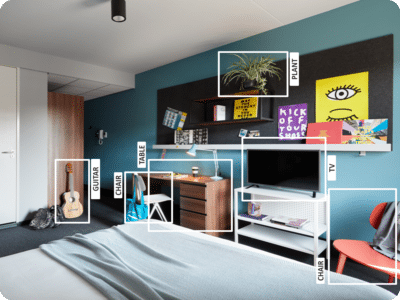## Applications Of Object Detection

### Facial Recognition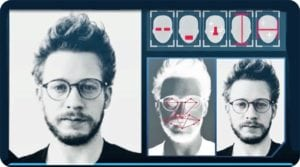A deep learning facial recognition system called the “DeepFace” has been developed by a group of researchers from Facebook, which identifies human faces in a digital image very effectively. Google uses its own facial recognition system in Google Photos, which automatically segregates all the photos based on the person in the image. There are various components involved in facial recognition, such as eyes, noses, mouths, and eyebrows.

### People Counting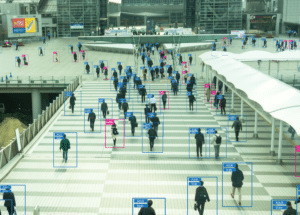Object detection can also be used for people counting. It is used for analyzing store performance or crowd statistics during festivals. These tend to be more difficult as people move out of the frame quickly.

It is a very important application, as during crowd gatherings, this feature can be used for multiple purposes.

### Industrial Quality Check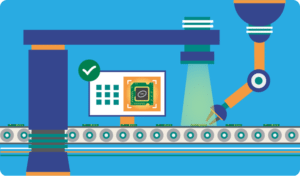Object detection is also used in industrial processes to identify products. Finding a specific object through visual inspection is a basic task that is involved in multiple industrial processes like sorting, inventory management, machining, quality management, packaging, etc.

Inventory management can be very tricky, as items are hard to track in real time.Automatic object counting and localization allows for improving inventory accuracy.

### Self Driving Cars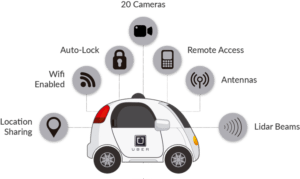Self-driving cars are the future; there’s no doubt about that. But the working behind it is very tricky, as it combines a variety of techniques to perceive their surroundings, including radar, laser light, GPS, odometry, and computer vision.

Advanced control systems interpret sensory information to identify appropriate navigation paths as well as obstacles, and once the image sensor detects any sign of a living being in its path, it automatically stops. This happens at a very fast rate and is a big step towards driverless cars.

### Security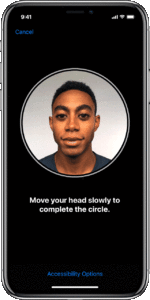Object detection plays a very important role in Security. Be it face ID of Apple or the retina scan used in all the sci-fi movies.

It is also used by the government to access security feeds and match it with their existing database to find any criminals or to detect the robbers’ vehicle.

The applications are limitless.

## Object Detection Workflow

Every object detection algorithm has a different way of working, but they all work on the same principle.

Feature Extraction: They extract features from the input images at hand and use these features to determine the class of the image. Be it through MatLab, Open CV, Viola Jones, or deep learning.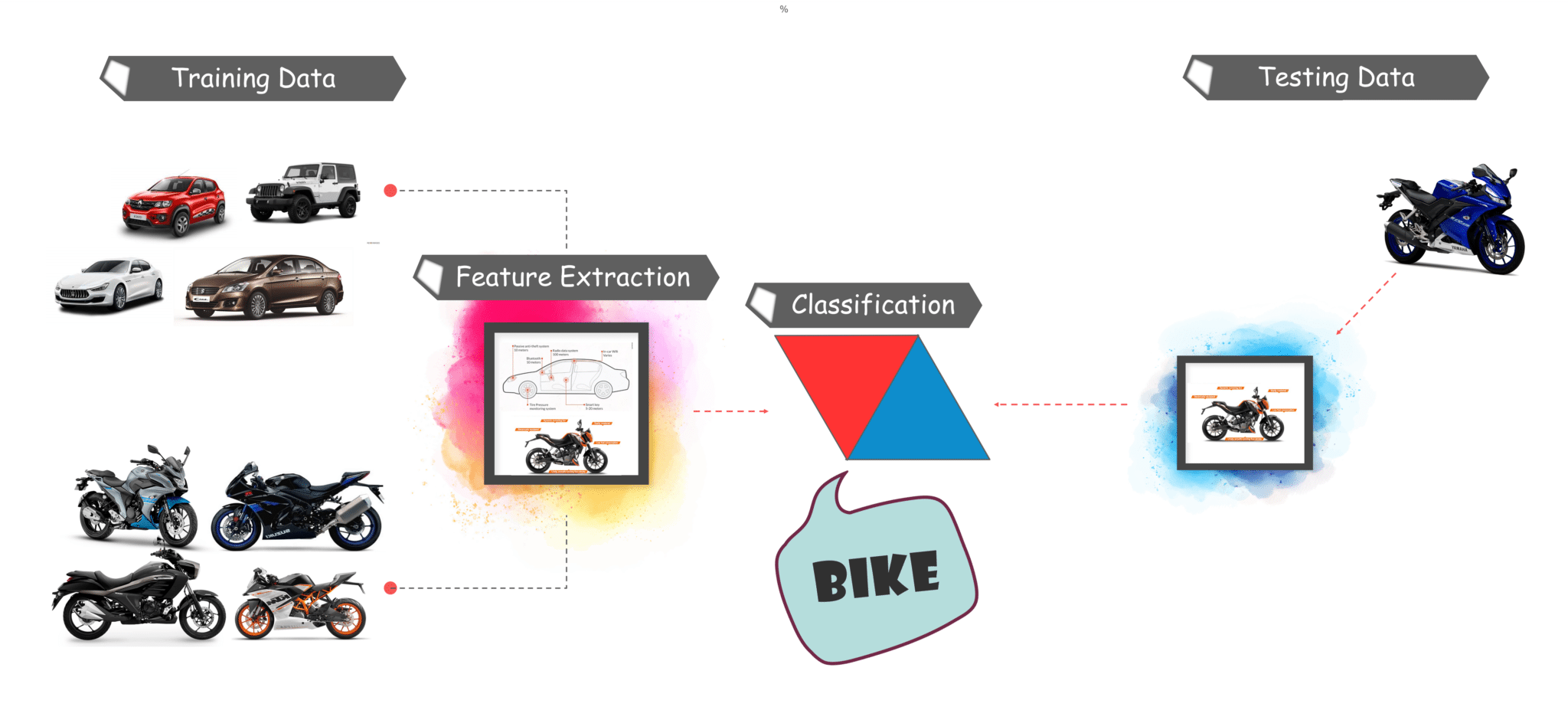that you have understand the basic workflow of object detection, let’s move along to the tutorial and understand what Tensorflow is and what its components are.

## What Is TensorFlow?

Tensorflow is Google’s open source machine learning framework for dataflow programming across a range of tasks. Nodes in the graph represent mathematical operations, while the graph edges represent the multi-dimensional data arrays (tensors) communicated between them.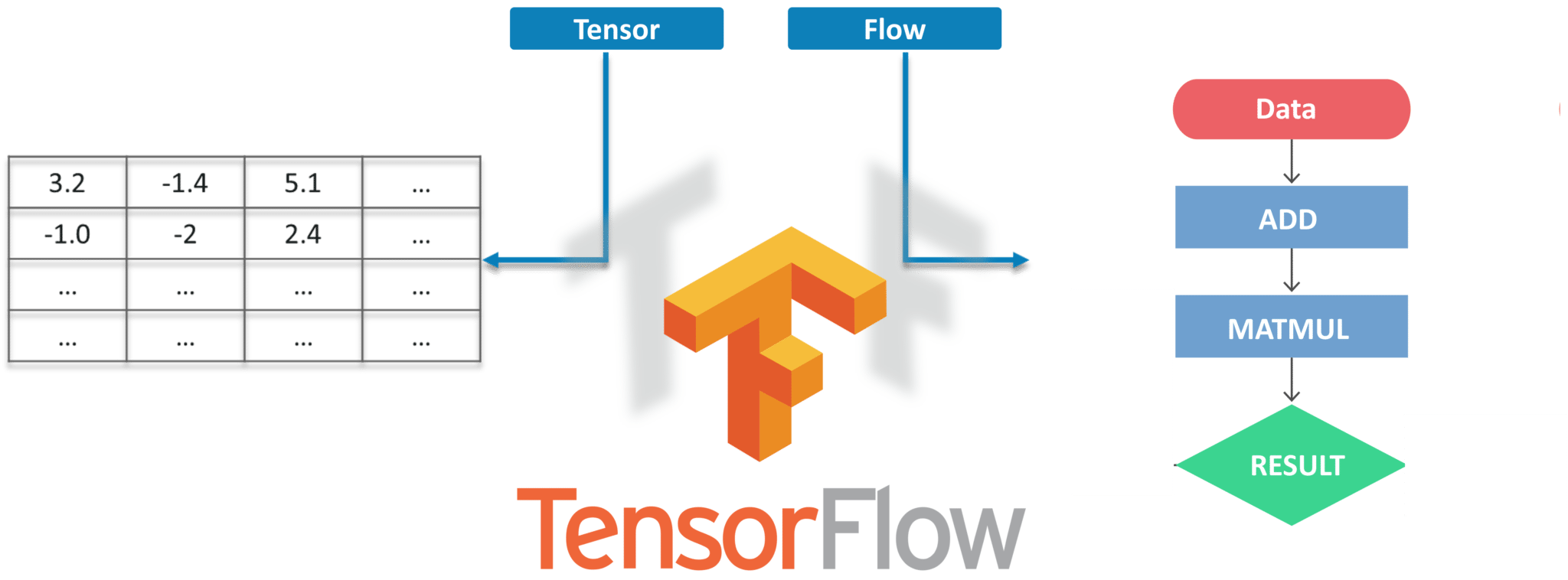Tensors are just multidimensional arrays, an extension of 2-dimensional tables of data with a higher dimension. There are many features of Tensorflow that make it appropriate for deep learning. So, without further ado, let’s see how we can implement Object Detection using Tensorflow.

## Object Detection Tutorial

### Prerequisites

Before working on the demo, let’s have a look at the prerequisites:

### Setting up the Environment

To Download TensorFlow and TensorFlow GPU, you can use pip or conda commands:

``````# For CPU
pip install tensorflow
# For GPU
pip install tensorflow-gpu``````

For all the other libraries, we can use pip or conda to install them. The code is provided below:

``````pip install --user Cython
pip install --user contextlib2
pip install --user pillow
pip install --user lxml
pip install --user jupyter
pip install --user matplotlib``````

Next, we have Protobuf: Protocol Buffers (Protobuf) is Google’s language-neutral, platform-neutral, extensible mechanism for serializing structured data. Think of it as XML, but smaller, faster, and simpler. You need to Download Protobuf version 3.4 or above for this demo and extract it.

For simplicity, we are going to keep “models” and “protobuf” under one folder named "Tensorflow."

Next, we need to go inside the Tensorflow folder and then inside the research folder and run protobuf from there using this command:

``"path_of_protobuf's bin"./bin/protoc object_detection/protos/``

To check whether this worked or not, you can go to the protos folder inside models>object_detection>protos and there, you can see that for every proto file, there’s one python file created.

### Main Code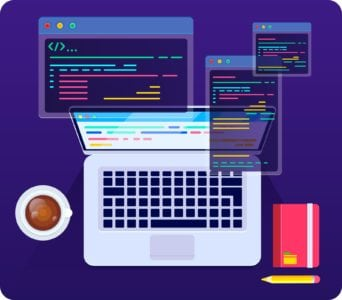After the environment is set up, you need to go to the “object_detection” directory and create a new python file. You can use Spyder or Jupyter to write your code.

First of all, we need to import all the libraries.

``````import numpy as np
import os
import six.moves.urllib as urllib
import sys
import tarfile
import tensorflow as tf
import zipfile

from collections import defaultdict
from io import StringIO
from matplotlib import pyplot as plt
from PIL import Image

sys.path.append("..")
from object_detection.utils import ops as utils_ops

from utils import label_map_util

from utils import visualization_utils as vis_util``````

Next, we will download the model, which is trained on the COCO dataset. COCO stands for Common Objects in Context, and this dataset contains around 330K labeled images. The model selection is important because you need to make a tradeoff between speed and accuracy. Depending on your requirement and the system memory, the correct model must be selected.

models>research>object_detection>g3doc>detection_model_zoo” contains all the models with different speed and accuracy (mAP).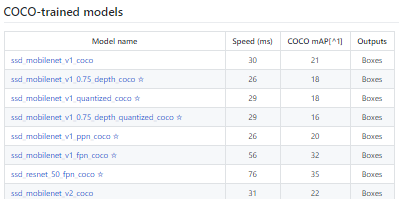Next, we provide the required model and the frozen inference graph generated by Tensorflow.

``````MODEL_NAME = 'ssd_mobilenet_v1_coco_2017_11_17'
MODEL_FILE = MODEL_NAME + '.tar.gz'

PATH_TO_CKPT = MODEL_NAME + '/frozen_inference_graph.pb'

PATH_TO_LABELS = os.path.join('data', 'mscoco_label_map.pbtxt')

NUM_CLASSES = 90``````

This code will download that model from the internet and extract the frozen inference graph of that model.

``````opener = urllib.request.URLopener()
tar_file = tarfile.open(MODEL_FILE)
for file in tar_file.getmembers():
file_name = os.path.basename(file.name)
if 'frozen_inference_graph.pb' in file_name:
tar_file.extract(file, os.getcwd())

detection_graph = tf.Graph()
with detection_graph.as_default():
od_graph_def = tf.GraphDef()
with tf.gfile.GFile(PATH_TO_CKPT, 'rb') as fid:
od_graph_def.ParseFromString(serialized_graph)
tf.import_graph_def(od_graph_def, name='')``````

Next, we are going to load all the labels

``````label_map = label_map_util.load_labelmap(PATH_TO_LABELS)
categories = label_map_util.convert_label_map_to_categories(label_map, max_num_classes=NUM_CLASSES, use_display_name=True)
category_index = label_map_util.create_category_index(categories)
``````

Now we will convert the images' data into a numPy array for processing.

``````def load_image_into_numpy_array(image):
(im_width, im_height) = image.size
return np.array(image.getdata()).reshape(
(im_height, im_width, 3)).astype(np.uint8)``````

The path to the images for the testing purpose is defined here. We have a naming convention “image[i]” for i in (1 to n+1), n being the number of images provided.

``````PATH_TO_TEST_IMAGES_DIR = 'test_images'
TEST_IMAGE_PATHS = [ os.path.join(PATH_TO_TEST_IMAGES_DIR, 'image{}.jpg'.format(i)) for i in range(1, 8) ]``````

This code runs the inference for a single image where it detects the objects, make boxes, and provides the class and the class score of that particular object.

``````def run_inference_for_single_image(image, graph):
with graph.as_default():
with tf.Session() as sess:
# Get handles to input and output tensors
ops = tf.get_default_graph().get_operations()
all_tensor_names = {output.name for op in ops for output in op.outputs}
tensor_dict = {}
for key in [
'num_detections', 'detection_boxes', 'detection_scores',
]:
tensor_name = key + ':0'
if tensor_name in all_tensor_names:
tensor_dict[key] = tf.get_default_graph().get_tensor_by_name(
tensor_name)
# The following processing is only for single image
detection_boxes = tf.squeeze(tensor_dict['detection_boxes'], )
# Reframe is required to translate mask from box coordinates to image coordinates and fit the image size.
real_num_detection = tf.cast(tensor_dict['num_detections'], tf.int32)
detection_boxes = tf.slice(detection_boxes, [0, 0], [real_num_detection, -1])
image_tensor = tf.get_default_graph().get_tensor_by_name('image_tensor:0')

# Run inference
output_dict = sess.run(tensor_dict,
feed_dict={image_tensor: np.expand_dims(image, 0)})

# all outputs are float32 numpy arrays, so convert types as appropriate
output_dict['num_detections'] = int(output_dict['num_detections'])
output_dict['detection_classes'] = output_dict[
'detection_classes'].astype(np.uint8)
output_dict['detection_boxes'] = output_dict['detection_boxes']
output_dict['detection_scores'] = output_dict['detection_scores']
return output_dict``````

Our final loop, which will call all the functions defined above, will run the inference on all the input images one by one, which will provide us the output of images in which objects are detected with labels and the percentage/score of that object being similar to the training data.

``````for image_path in TEST_IMAGE_PATHS:
image = Image.open(image_path)
# the array based representation of the image will be used later in order to prepare the
# result image with boxes and labels on it.
# Expand dimensions since the model expects images to have shape: [1, None, None, 3]
image_np_expanded = np.expand_dims(image_np, axis=0)
# Actual detection.
output_dict = run_inference_for_single_image(image_np, detection_graph)
# Visualization of the results of a detection.
vis_util.visualize_boxes_and_labels_on_image_array(
image_np,
output_dict['detection_boxes'],
output_dict['detection_classes'],
output_dict['detection_scores'],
category_index,
use_normalized_coordinates=True,
line_thickness=8)
plt.figure(figsize=IMAGE_SIZE)
plt.imshow(image_np)``````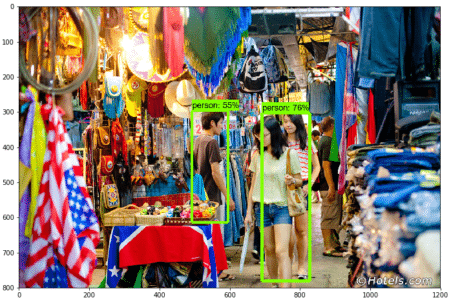Now, let’s see how we can detect objects in a live video feed.

## Live Object Detection Using Tensorflow

For this demo, we will use the same code, but we’ll tweak a few things. We are going to use OpenCV and the camera module to use the live feed of the webcam to detect objects.

Add the OpenCV library and the camera being used to capture images. Just add the following lines to the import library section.

``````import cv2
cap = cv2.VideoCapture(0)``````

We don’t need to load the images from the directory and convert it to numPy array, as OpenCV will take care of that for us.

Replace this:

``````for image_path in TEST_IMAGE_PATHS:
image = Image.open(image_path)
# the array based representation of the image will be used later in order to prepare the
# result image with boxes and labels on it.

With this:

``````while True:

We will not use matplotlib for final image show. Instead, we will use OpenCV for that as well. For that...

Remove this:

``````cv2.imshow('object detection', cv2.resize(image_np, (800,600)))
if cv2.waitKey(25) & 0xFF == ord('q'):
cv2.destroyAllWindows()
break``````

This code will use OpenCV that will, in turn, use the camera object initialized earlier to open a new window named “Object_Detection” of the size “800×600”. It will wait for 25 milliseconds for the camera to show images, otherwise, it will close the window.

### Final Code With All the Changes:

``````import numpy as np
import os
import six.moves.urllib as urllib
import sys
import tarfile
import tensorflow as tf
import zipfile

from collections import defaultdict
from io import StringIO
from matplotlib import pyplot as plt
from PIL import Image

import cv2
cap = cv2.VideoCapture(0)

sys.path.append("..")

from utils import label_map_util

from utils import visualization_utils as vis_util

MODEL_NAME = 'ssd_mobilenet_v1_coco_11_06_2017'
MODEL_FILE = MODEL_NAME + '.tar.gz'

# Path to frozen detection graph. This is the actual model that is used for the object detection.
PATH_TO_CKPT = MODEL_NAME + '/frozen_inference_graph.pb'

# List of the strings that is used to add correct label for each box.
PATH_TO_LABELS = os.path.join('data', 'mscoco_label_map.pbtxt')

NUM_CLASSES = 90

opener = urllib.request.URLopener()
tar_file = tarfile.open(MODEL_FILE)
for file in tar_file.getmembers():
file_name = os.path.basename(file.name)
if 'frozen_inference_graph.pb' in file_name:
tar_file.extract(file, os.getcwd())

detection_graph = tf.Graph()
with detection_graph.as_default():
od_graph_def = tf.GraphDef()
with tf.gfile.GFile(PATH_TO_CKPT, 'rb') as fid:
od_graph_def.ParseFromString(serialized_graph)
tf.import_graph_def(od_graph_def, name='')

categories = label_map_util.convert_label_map_to_categories(label_map, max_num_classes=NUM_CLASSES, use_display_name=True)
category_index = label_map_util.create_category_index(categories)

with detection_graph.as_default():
with tf.Session(graph=detection_graph) as sess:
while True:
# Expand dimensions since the model expects images to have shape: [1, None, None, 3]
image_np_expanded = np.expand_dims(image_np, axis=0)
image_tensor = detection_graph.get_tensor_by_name('image_tensor:0')
# Each box represents a part of the image where a particular object was detected.
boxes = detection_graph.get_tensor_by_name('detection_boxes:0')
# Each score represent how level of confidence for each of the objects.
# Score is shown on the result image, together with the class label.
scores = detection_graph.get_tensor_by_name('detection_scores:0')
classes = detection_graph.get_tensor_by_name('detection_classes:0')
num_detections = detection_graph.get_tensor_by_name('num_detections:0')
# Actual detection.
(boxes, scores, classes, num_detections) = sess.run(
[boxes, scores, classes, num_detections],
feed_dict={image_tensor: image_np_expanded})
# Visualization of the results of a detection.
vis_util.visualize_boxes_and_labels_on_image_array(
image_np,
np.squeeze(boxes),
np.squeeze(classes).astype(np.int32),
np.squeeze(scores),
category_index,
use_normalized_coordinates=True,
line_thickness=8)

cv2.imshow('object detection', cv2.resize(image_np, (800,600)))
if cv2.waitKey(25) 0xFF == ord('q'):
cv2.destroyAllWindows()
break``````

I hope you all enjoyed this article and now understand the power of Tensorflow and how easy it is to detect objects in images and live video feed. So, if you have read this, you are no longer a newbie on object detection and TensorFlow. Try out these examples and let me know if you face any challenges while deploying the code.

Topics:
tensorflow ,object detection ,tensorflow tutorial ,artificial intelligence ,tutorial ,machine learning models ,advanced driver assisstance systems

Comment (5)

Save
{{ articles.views | formatCount}} Views

Published at DZone with permission of Kislay Keshari , DZone MVB. See the original article here.

Opinions expressed by DZone contributors are their own.